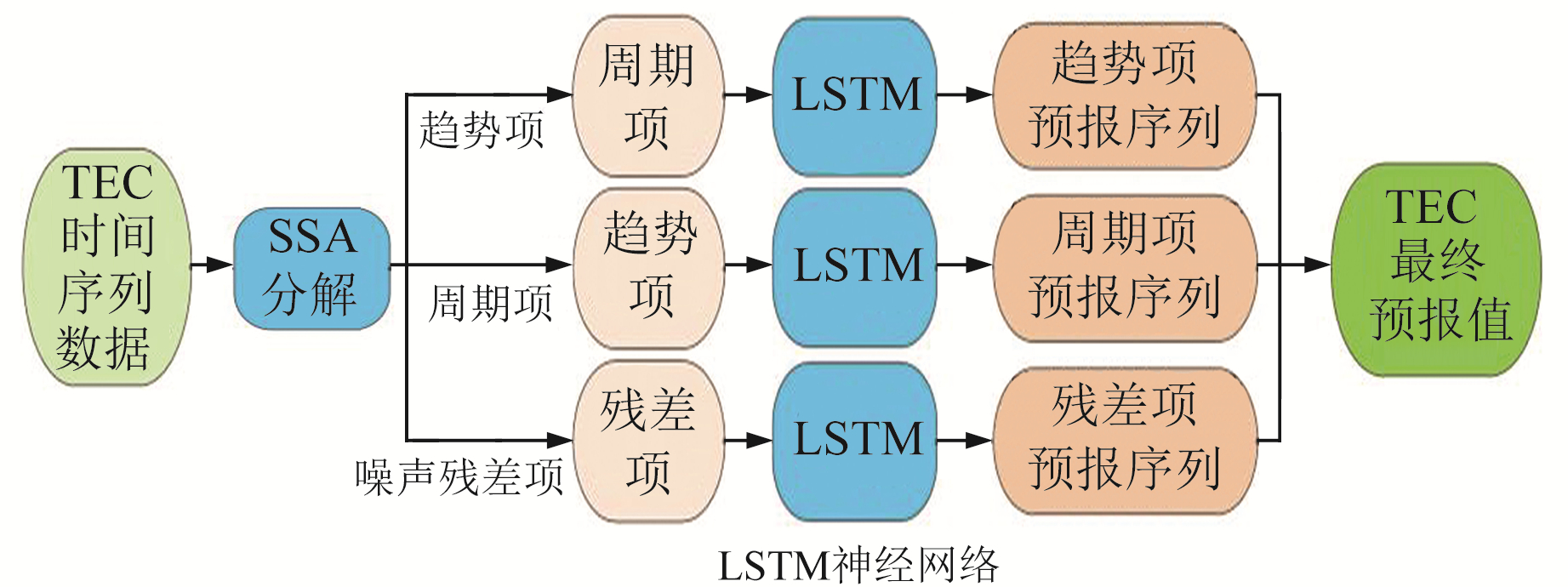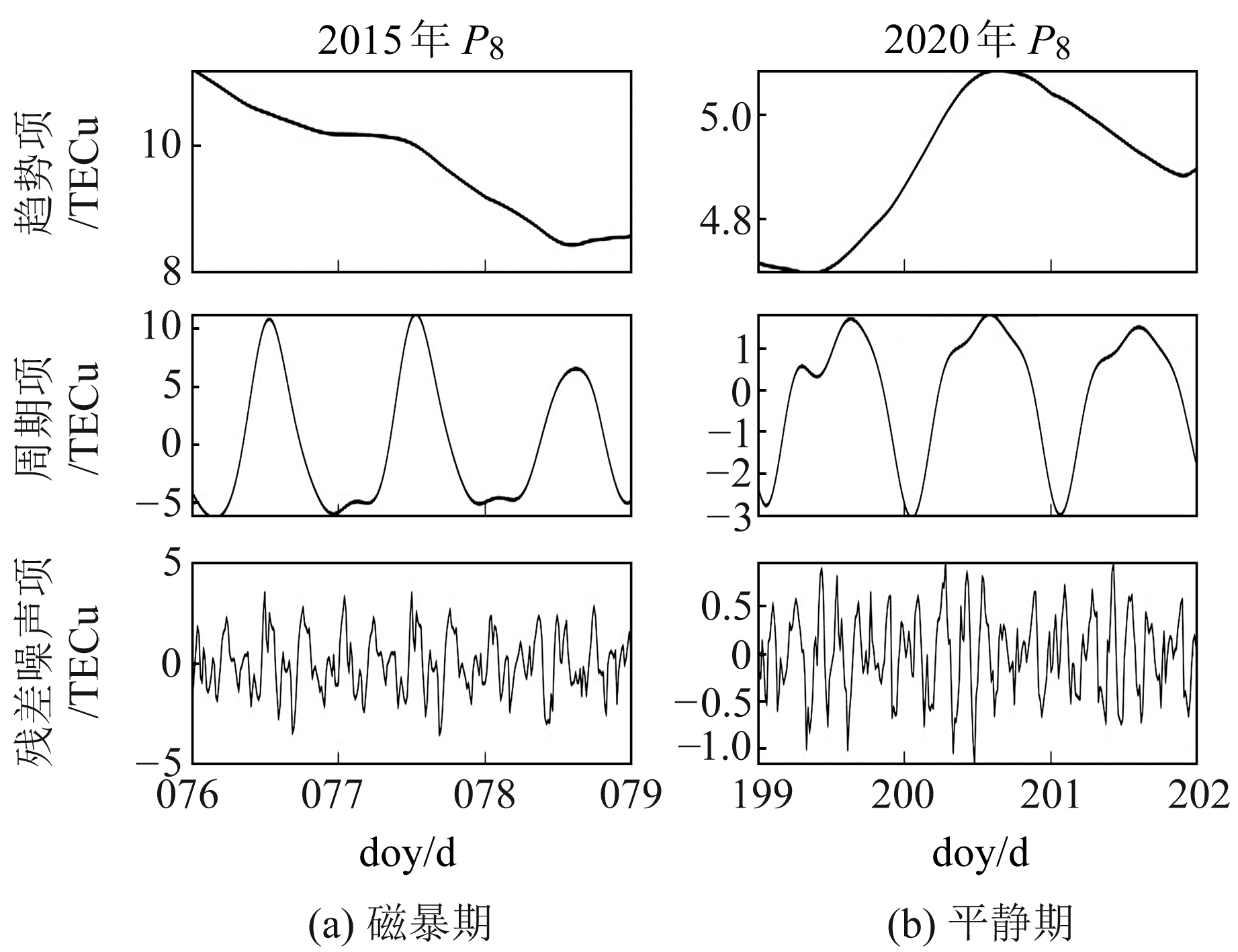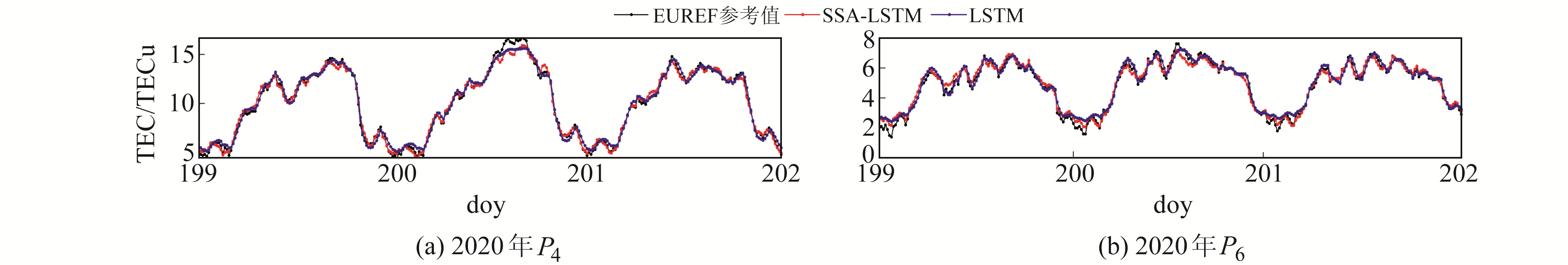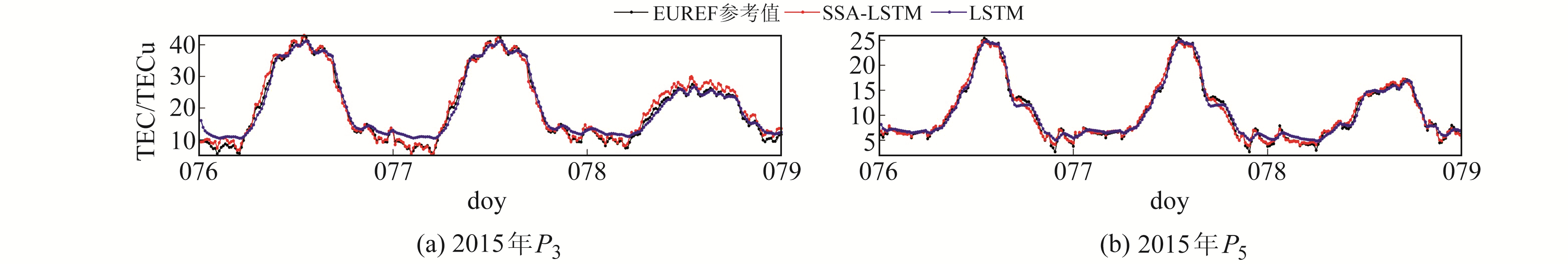﻿ 基于SSA-LSTM的短期电离层TEC组合预报模型文章快速检索 高级检索
 大地测量与地球动力学2022, Vol. 42Issue (6): 626-630  DOI: 10.14075/j.jgg.2022.06.014### 引用本文WU Han, HUANG Ling, LIU Lilong, et al. Short Term Prediction Model of Ionospheric TEC Based on SSA-LSTM[J]. Journal of Geodesy and Geodynamics, 2022, 42(6): 626-630.### Foundation support

Guangxi Natural Science Foundation, No. 2020GXNSFBA159033; Guangxi Science and Technology Base and Talent Project, No.AD19245060; Project of Guangxi Key Laboratory of Spatial Information and Geomatics, No. 19-050-11-24.

### Corresponding author

HUANG Ling, PhD, lecturer, majors in GNSS ionosphere and precise positioning, E-mail: linghuang@glut.edu.cn.

### 第一作者简介

WU Han, postgraduate, majors in GNSS space environment, E-mail: wh1491432235@163.com.

### 文章历史

1. 桂林理工大学测绘地理信息学院, 桂林市雁山街319号, 541006;
2. 广西空间信息与测绘重点实验室, 桂林市雁山街319号, 541006;
3. 武汉大学卫星导航定位技术研究中心, 武汉市珞喻路129号, 430079

1 模型算法原理 1.1 奇异谱分析理论

1) 构建轨迹矩阵X(嵌入)。

 $\begin{array}{c} \boldsymbol{X}=\left[\boldsymbol{X}_{1}, \boldsymbol{X}_{2}, \boldsymbol{X}_{3}, \cdots, \boldsymbol{X}_{K}\right]=\left(x_{i j}\right)_{i, j=1}^{M, K}= \\ {\left[\begin{array}{cccc} s_{0} & s_{1} & \cdots & s_{K} \\ s_{1} & s_{2} & \cdots & s_{K+1} \\ \vdots & \vdots & \ddots & \vdots \\ s_{M} & s_{M+1} & \cdots & s_{N} \end{array}\right]} \end{array}$ (1)

2) 奇异值分解(SVD)。

X可形成M阶矩阵A=XXT，$\sqrt{\lambda_{i}}$为矩阵X的奇异值, i=1, 2, 3, …, d, d=rank(X)。Ui为矩阵X的左奇异向量。设向量Vi为矩阵X的右奇异向量，$\boldsymbol{V}_{i}=\frac{\boldsymbol{X}^{\mathrm{T}} \boldsymbol{U}_{i}}{\sqrt{\lambda}}$。轨迹矩阵X可分解为若干个与其结构相同的初等矩阵$\boldsymbol{E}_{i}=\sqrt{\lambda} \boldsymbol{U}_{i} \boldsymbol{V}_{i}^{\mathrm{T}}$。

3) 分组。

4) 对角平均化(重构)。

 ${z_i} = \left\{ {\begin{array}{*{20}{l}} {\frac{1}{i}\sum\limits_{m = 1}^i {z_{m, i - m + 1}^*} , 1 \le i \le {M^*}}\\ {\frac{1}{{{M^*}}}\sum\limits_{m = 1}^{{L^*}} {z_{m, i - m + 1}^*} , {M^*} < i \le {K^*}}\\ {\frac{1}{{N - i + 1}}\sum\limits_{m = i - {K^*} + 1}^{N - {K^*} + 1} {z_{m, i - m + 1}^*} , {K^*} < i \le N} \end{array}} \right.$ (2)
1.2 LSTM

LSTM模型神经元通过添加3个“门”(输入门、遗忘门、输出门)的结构来替换RNN神经元，以改进长期序列的预测：输入门通过确定在单元格状态中需要记住的新信息来处理输入；遗忘门从单元格状态中删除不重要的信息；输出门提取存储在单元格状态中的信息，实现选择记忆反馈的误差函数随梯度下降的修正参数，从而实现时间上的记忆功能，并防止梯度消失[9-10]。其网络神经元结构如图 1所示，Ott分别为t时刻神经元经过输出门的输出结果和当前输入的单元状态；ht-1为隐藏层状态；Ct-1为上一时刻的单元状态；Ct为更新后的神经元状态信息；σ为sigmoid激活函数，值域(0, 1)；tanh为双曲正切激活函数。图 1 LSTM模型神经元网络结构 Fig. 1 Neural network structure of LSTM model
1.3 SSA-LSTM模型图 2 SSA-LSTM模型示意图 Fig. 2 Schematic diagram of SSA-LSTM model
2 实验及分析 2.1 实验数据处理图 3 SSA重构分量 Fig. 3 Component reconstructed with SSA
2.2 预报结果分析 2.2.1 地磁活动情况

Dst(disturbance storm time)是研究地磁暴及其相关现象的重要指标。本文采用ICSU-WDS数据中心(http://wdc.kugi.kyoto-u.ac.jp/wdc/Sec3.html)提供的地磁指数Dst，参考地磁暴强度等级标准文件(GB/T 31160-2014)和NOAA空间天气预报中心等级标准，得到Dst值与磁暴等级如表 1所示。表 1 磁暴等级划分 Tab. 1 Magnetic explosion classification表 2 预报时段的Dst指数 Tab. 2 Dst index of forecast periods
2.2.2 不同地磁活动条件下预报模型改进精度分析

 $P = 1 - \frac{{\left| {{\rm{te}}{{\rm{c}}_p} - {\rm{te}}{{\rm{c}}_r}} \right|}}{{{{{\mathop{\rm tec}\nolimits} }_r}}}$ (3)
 ${\rm{RMSE}} = \sqrt {\frac{1}{n}\sum\limits_{i = 1}^n {{{\left( {{{{\mathop{\rm tec}\nolimits} }_p} - {\rm{te}}{{\rm{c}}_r}} \right)}^2}} }$ (4)图 4 地磁平静期2种模型在P4、P6格网点的TEC预报结果对比 Fig. 4 Comparison of the prediction results of the two models during the geomagnetic calm period at P4 and P6 grid networks图 5 地磁平静期2种模型在P4、P6格网点的预报结果误差 Fig. 5 Errors of the prediction results of the two models during the geomagnetic calm period at P4 and P6 grid networks图 6 地磁活跃期2种模型在P3、P5格网点的TEC预报结果对比 Fig. 6 Comparison of the prediction results of the two models during the geomagnetic explosion period at P3 and P5 grid networks图 7 地磁活跃期2种模型在P3、P5格网点的预报结果误差 Fig. 7 Errors of the prediction results of the two models during the geomagnetic explosion period at P3 and P5 grid networks表 3 2种模型3 d预报结果RMSE百分比统计 Tab. 3 Statistics of RMSE percentage of 3-day prediction results of the two models图 8 2种模型在不同地磁活动情况下TEC预报结果的相对精度P和RMSE均值 Fig. 8 Results of the relative accuracy P and RMSE of TEC forecast results of the two models for different geomagnetic periods
3 结语

  Vautard R, Ghil M. Singular Spectrum Analysis in Nonlinear Dynamics, with Applications to Paleoclimatic Time Series[J]. Physica D: Nonlinear Phenomena, 1989, 35(3): 395-424 DOI:10.1016/0167-2789(89)90077-8 (0)  徐克红, 程鹏飞, 文汉江. 太阳黑子数时间序列的奇异谱分析和小波分析[J]. 测绘科学, 2007, 32(6): 35-38 (Xu Kehong, Cheng Pengfei, Wen Hanjiang. Singular Spectrum Analysis and Wavelet Analysis on Time Series of Sunspot[J]. Science of Surveying and Mapping, 2007, 32(6): 35-38) (0)  Zhou S K, Reuckert D, Fichtinger G. Handbook of Medical Image Computing and Computer Assisted Intervention[M]. Salt Lake: Academic Press, 2020 (0)  Al-Jabery K K, Obafemi-Ajayi T, Olbricht G R, et al. Computational Learning Approaches to Data Analytics in Biomedical Applications[M]. Salt Lake: Academic Press, 2020 (0)  吉长东, 王强, 王贵朋, 等. 深度学习LSTM模型的电离层总电子含量预报[J]. 导航定位学报, 2019, 7(3): 76-81 (Ji Changdong, Wang Qiang, Wang Guipeng, et al. TEC Prediction of Ionosphere Based on Deep Dearning LSTM Model[J]. Journal of Navigation and Positioning, 2019, 7(3): 76-81) (0)  王鹏程, 郭金运, 王方建, 等. 基于奇异谱分析的汶川地震震前地倾斜数据分析[J]. 地球物理学进展, 2020, 35(2): 467-474 (Wang Pengcheng, Guo Jinyun, Wang Fangjian, et al. Singular Spectrum Analysis on Ground Tilt Data before Wenchuan Earthquake[J]. Progress in Geophysics, 2020, 35(2): 467-474) (0)  卢辰龙, 匡翠林, 张晋升, 等. 组合SSA与ARMA模型预报电离层TEC[J]. 大地测量与地球动力学, 2014, 34(6): 44-49 (Lu Chenlong, Kuang Cuilin, Zhang Jinsheng, et al. Predicting Ionosphere TEC with the Combination of Singular Spectrum Analysis and ARMA Model[J]. Journal of Geodesy and Geodynamics, 2014, 34(6): 44-49) (0)  Vautard R, Yiou P, Ghil M. Singular-Spectrum Analysis: A Toolkit for Short, Noisy Chaotic Signals[J]. Physica D: Nonlinear Phenomena, 1992, 58(1-4): 95-126 DOI:10.1016/0167-2789(92)90103-T (0)  Chimsuwan P, Supnithi P, Phakphisut W, et al. Construction of LSTM Model for Total Electron Content(TEC) Prediction in Thailand[C]. International Conference on Electrical Engineering/Electronics, Computer, Telecommunications and Information Technology(ECTI-CON), Chiang Mai, 2021 (0)  Cheng N, Kuo A. Using Long Short-Term Memory(LSTM) Neural Networks to Predict Emergency Department Wait Time[J]. Studies in Health Technology and Informatics, 2020, 270: 1 425-1 426 (0)
Short Term Prediction Model of Ionospheric TEC Based on SSA-LSTM
WU Han1,2HUANG Ling1,2LIU Lilong1,2     HUANG Liangke1,2     ZHANG Hongping3
1. College of Geomatics and Geoinformation, Guilin University of Technology, 319 Yanshan Street, Guilin 541006, China;
2. Guangxi Key Laboratory of Spatial Information and Geomatics, 319 Yanshan Street, Guilin 541006, China;
3. GNSS Research Centre, Wuhan University, 129 Luoyu Road, Wuhan 430079, China
Abstract: Aiming at the high noise, nonlinear and non-stationary dynamic characteristics of ionospheric total electron content(TEC) time series, we construct an improved short-term ionospheric combined prediction model based on singular spectrum analysis(SSA) and long short term memory(LSTM) neural network model, to realize the model's ionospheric TEC prediction during magnetic storms and magnetic quiet periods and analyze its accuracy. The results show that the relative accuracy of model is 91.17% and 95.46% respectively, which is 4.92 percent and 3.17 percent higher than that of single LSTM model, during the period of magnetic explosion and magnetic calm.
Key words: long-short term memory neural network; singular spectrum analysis(SSA); geomagnetic activity(SSA); ionospheric TEC prediction